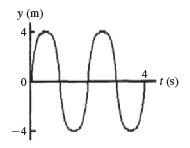# Problem: What is the frequency of the wave shown in the figure?a. 2 Hz. b. 4 Hz. c. 1 Hz. d. It cannot be determined from the given information. e. 0.5 Hz.

###### FREE Expert Solution

Frequency:

$\overline{){\mathbf{f}}{\mathbf{=}}\frac{\mathbf{1}}{\mathbf{T}}}$

80% (7 ratings)###### Problem Details

What is the frequency of the wave shown in the figure?a. 2 Hz.

b. 4 Hz.

c. 1 Hz.

d. It cannot be determined from the given information.

e. 0.5 Hz.

Frequently Asked Questions

What scientific concept do you need to know in order to solve this problem?

Our tutors have indicated that to solve this problem you will need to apply the Waves on a String concept. You can view video lessons to learn Waves on a String. Or if you need more Waves on a String practice, you can also practice Waves on a String practice problems.

What professor is this problem relevant for?

Based on our data, we think this problem is relevant for Professor Dubey's class at UCF.